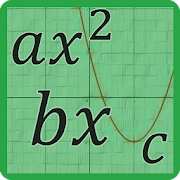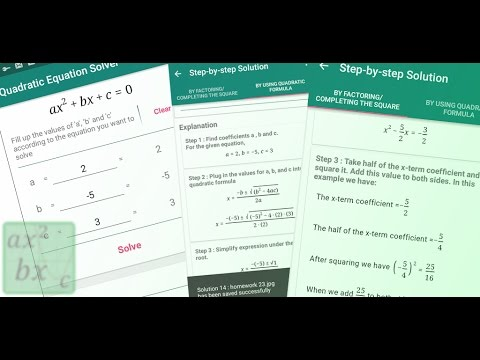# Quadratic Equation Solver with Steps and GraphsEveryone
807
Contains AdsThis is a smart application which solves quadratic equations or formulas and gives you the step-by-step solution. Unlike most of the other apps, this app is featured with both "Quadratic Formula" and "Completing the square" methods.

★ Capable of generating graphs for a given equation.

★ Both "Quadratic Formula" and "Completing the square" methods available.

★ Ability to save step-by-step solution as an image.

★ User friendly interface with Material design.

★ Decimal and fractional number inputs.

★ Decimal and fractional number output.

★ Handles imaginary numbers.

★ Each variable input is a simple calculator supporting the following operators ( *, /, +, -).

★ Lightweight.

NOTE : Quadratic equations are of the form ax2+bx+c=0 where a, b and c are real numbers and "a" should not be equal to zero. Quadratic equations have two solutions. It is possible that one solution may repeat. You can calculate quadratic equations by Completing the square and by Quadratic Formula.

Solving by Competing the Square

• Keep all terms containing x on one side. Move the constant to the right.

• Get ready to create a perfect square on the left. Balance the equation.

• Take half of the x-term coefficient and square it. Add this value to both sides.

• Simplify and write the perfect square on the left.

• Take the square root of both sides. Be sure to allow for both plus and minus.

• Solve for x.

Solving by Quadratic Formula

The solutions of some quadratic equations are not rational, and cannot be factored. For such equations, the most common method of solution is the quadratic formula. The quadratic formula can be used to solve ANY quadratic equation.
Read more
Collapse

Review Policy
4.6
807 total
5
4
3
2
1
Loading...

## What's New

V 1.4.2
+ Performance improvements
+ Released the PRO version. You can get it for FREE until 5th-Sep-2018.

V 1.3.0
+ New settings screen for app settings

V 1.2.7
+ Landscape mode is supported.
+ Performance improvements
+ New languages added [Spanish, Russian, Portuguese]
+ Ads will be displayed (but if you want, you can request the ad-free version for free)
Read more
Collapse

## Additional Information

Updated
September 2, 2018
Size
2.9M
Installs
100,000+
Current Version
1.4.2
Requires Android
4.1 and up
Content Rating
Everyone
Permissions
Offered By
K. Dev
Developer
Kasun Dissanayake, Etaweeragollewa, Madawachchiya, Sri Lanka
©2019 GoogleSite Terms of ServicePrivacyDevelopersArtistsAbout Google|Location: United StatesLanguage: English (United States)
By purchasing this item, you are transacting with Google Payments and agreeing to the Google Payments Terms of Service and Privacy Notice.